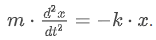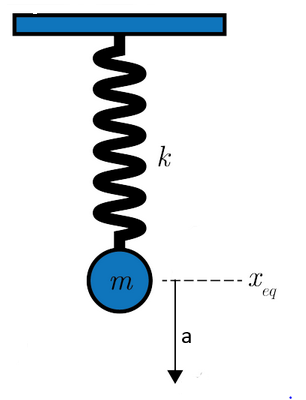# 3.2.4 Worked example 3.1

Course subject(s) Module 3: Waves

In this worked example we will show that the general solution x(t)=Asin(ωt+ϕ) for a mass spring system is a solution to the differential equation that follows from the force balance, which is given by:This solution is also referred to as the equation of motion of a harmonic oscillator.In the equation of motion:

•  x is the position of the mass with respect to the equilibrium position.
• A is the amplitude of the sinusoid, which is the maximum deviation from the equilibrium position.
• ω is the angular frequency at which the mass oscillates.
• ϕ is the phase deviation, which causes a nonzero starting point.

This worked example provides a framework to show that the equation of motion presented above is indeed a solution to the differential equation for the harmonic oscillator and to determine under which condition this is true.

In this exercise, you can write ω as omega and ϕ as phi.